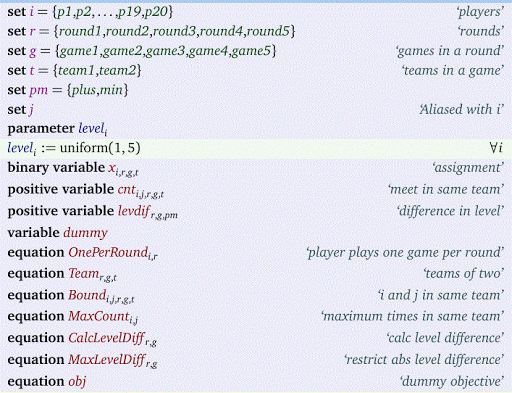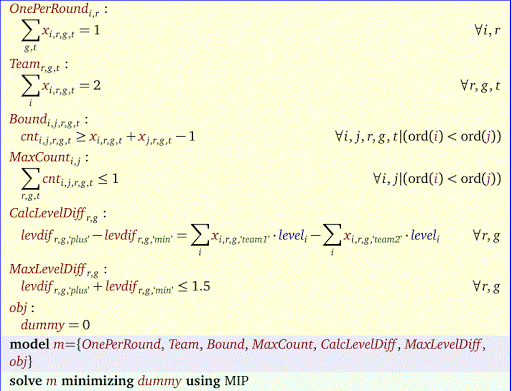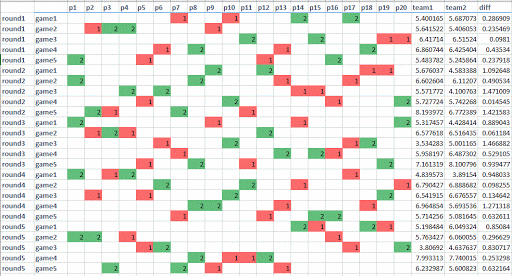## Monday, June 20, 2016

### More tennis schedulingIn this post another tennis-scheduling problem is posted. In this case we deal with 20 players doing doubles in 5 rounds. That means teams of 2 players, 4 players per game, and 5 games per round.

##### Restriction 1

The first restriction is that a player $$i$$ and player $$j$$ can be team-members only once. I.e. they can play together zero or one time. There is no restriction on how many times player $$i$$ can meet player $$j$$ as opponent.

##### Restriction 2

Each player has a rating or level between 1 and 5. The level of a team is the sum of the ratings of the two players.  We don’t want the level of two opposing teams to differ by more than 1.5.

##### The ModelThe main variable is $$x_{i,r,g,t}$$ which indicates if player $$i$$ is playing in round $$r$$, game $$g$$ in team $$t$$.

The level difference restriction:$\max_{i<j} \sum_{r,g,t} x_{i,r,g,t} x_{j,r,g,t} \le 1$ is not completely trivial to linearize. The idea is to linearize the inner product by introducing additional variables $$cnt_{i,j,r,g,t}$$. Then we just sum these. Note that $$cnt_{i,j,r,g,t}$$ can be considered a binary variable. I relaxed that here to a positive continuous variable (you may verify this is allowed here).

The difference in level of two opposing teams is implemented using a standard variable splitting technique. We used random player levels.The final solution is:It is noted that instead of a bound on the level difference, we also could minimize this quantity (allowing for even better matchups where possible).